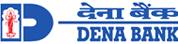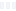• # Compound Interest Formula

Understanding the concept of compound interest, its formula, and how it is calculated is useful because it is the basis of how interest is calculated for your stock market investments, fixed deposits, recurring deposits, etc. It can help you determine how much your return on investment will be, thereby helping you to plan your savings even better. Retail loans such as home loans and vehicle loans also use the compound interest formula so understanding this will give you a better picture of how much interest you will be paying over the years. Here's an example of how it grows year by year:

• Year 1 - You earn interest on your Principal amount.
• Year 2 - You earn interest on the amount which is the Principal + Interest of Year 1.
• Year 3 - You earn interest on the amount which is the Principal + Interest of Year 1 + Interest of Year 2.

## Types of Compound Interest

There are generally two types of compound interest used.

• Periodic Compounding - Under this method, the interest rate is applied at intervals and generated. This interest is added to the principal. Periods here would mean annually, bi-annually, monthly, or weekly.
• Continuous Compounding - This method uses a natural log-based formula and calculates interest at the smallest possible interval. This interest is added back to the principal. This can be equalled to the constant rate of growth for all natural growth. This figure was born out of physics. It uses Euler’s number which is a famous irrational number which is known to more than 1 trillion digits of accuracy. Euler’s number is denominated by the letter “E”.

## Periodic Compound Interest Formula Overview

There are two formulas you can use to calculate compound interest, depending on what result you wish to find out. You can find out the following:

• The total value of the deposit.
• The total compound interest earned.

### Value of the Deposit

Formulas can be a deterrent to many. If you aren’t savvy with math, your eyes turn away from these codes or just skip them altogether. But once it’s explained, it’s pretty simple to understand. To calculate the total value of your deposit, the formula is as follows:

P (1+ i/n)nt

P = Principal invested.

i = Nominal Rate of Interest.

n = Compounding Frequency or number of compounding periods in a year.

t = Time, meaning the length of time the interest is applicable, generally in years.

Simply put, you calculate the interest rate divided by the number of times in a year the compound interest is generated. For instance, if your bank compounds interest quarterly, there are 4 quarters in a year, so n = 4. This result must be multiplied to the power of the deposit period. For example, if your deposit is for 10 years, t = 10. This whole result should be multiplied by the principal you invested. The result generated will equal the total accumulated value of your deposit. You can find out how much your deposit is worth currently after accumulating interest.

### Total Compound Interest Earned

To find out how much interest was earned, you can use the following formula for Compound Interest

P[(1+ i/n))nt-1]

## Compound Interest Equation and Calculation

To understand the compound interest equation further, we can break it down in simpler terms. If you decide to invest in a fixed deposit with compound interest, this is how you will earn interest every year.

Period Deposit Balance
Investment P
Year 1 P + iP
Year 2 (P+ iP) + i(P+iP)

To collapse this formula, we can pull out factors of (1+i). Simply substitute iP with (1+i) to get the following:

Period Deposit Balance
Investment P
Year 1 P(1+i)
Year 2 P(1+i)2
Year 3 P(1+i)3

## Formula for Annual Compound Interest

To calculate the compound interest for a number of years together, we need to multiply P(1+i) to the power of the number of years of the deposit. So we end up with this formula:

P (1+ i/n)n

This formula can be used to calculate compound interest that is compounded annually. This means you receive interest only once a year. It is added to your principal, and you continue to earn interest on the new amount.

## Half-Yearly, Quarterly, Monthly Compound Interest Formula

If you are earning interest multiple times in a year, you need to factor in this number into the equation. So the formula generated is:

P (1+ i/n)nt

This formula can also be used for instances where the interest is compounded once every two years. In this case, n = 0.5, as each year is calculated as half.

## Examples of Compound Interest

For example, Rs. 10,000 is invested in a fixed deposit for 10 years. The interest is compounded every quarter which means 4 times in a year. The interest paid by the bank is 5%. To find out your nominal rate of interest, you need to divide 5 by 100 which equals 0.05. Now, we look at the formula and substitute the letters with the relevant numbers.

### Calculating the Total Value of the Deposit

P (1+ i/n)nt

Step 1: 10,000 (1+0.05/4)4x10

Step 2: 10,000(1+0.0125)40

Step 3: 10,000 (1.0125)40

Step 4: 10,000 (1.64361946349)

Step 5: 16436.1946349

We can round of this total to Rs. 16,436.19. So the compound interest earned after 10 years is Rs. 6,436.19.

### Calculating the Interest Earned

We can also arrive at this figure using the formula for compound interest earned. We can substitute the numbers for letters as seen below:

P[(1+ i/n)nt -1]

Step 1: 10,000 [(1+0.05/4)4x10 -1]

Step 2: 10,000 [(1+0.0125)40-1]

Step 3: 10,000 [(1.0125)40-1]

Step 4: 10,000 [(1.64361946349) -1]

Step 5: 10,000 (0.664361946349

Step 5: 6436.1946349

We can now add this interest earned to the principal amount to find out the value of the deposit. The maturity value will be Rs. 16,436.19.

The earnings through compound interest can be demonstrated with the following graph.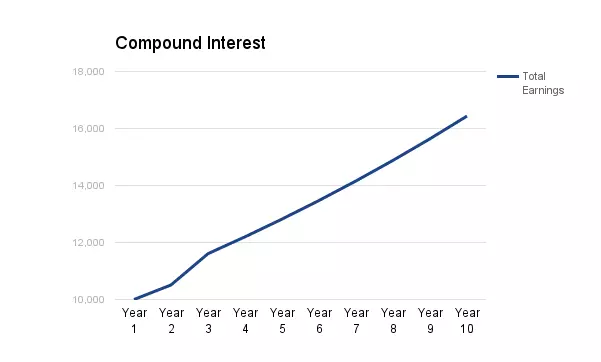## Simple Interest Formula vs. Compound Interest Formula

To demonstrate the difference between simple interest and compound interest, let’s take for example two fixed deposits. Both deposits are of Rs. 10,000 for 10 years. The interest offered on Deposit 1 is 5% compound interest. The interest offered on Deposit 2 is 5% simple interest. The interest is calculated annually on both deposits.

Period Deposit 1 - Compound Interest Deposit 2 - Simple Interest Difference
Year 1 Rs. 500 Rs. 500 Rs. 0
Year 2 Rs. 1,025.00 Rs. 1,000 Rs. 25
Year 3 Rs. 1,576.25 Rs. 1,500 Rs. 76.25
Year 4 Rs. 2,115.06 Rs. 2,000 Rs. 115.06
Year 5 Rs. 2,762.82 Rs. 2,500 Rs. 762.82
Year 6 Rs. 3,400.96 Rs. 3,000 Rs. 400.96
Year 7 Rs. 4,071.00 Rs. 3,500 Rs. 571.00
Year 8 Rs. 4,774.55 Rs. 4,000 Rs. 774.55
Year 9 Rs. 5,513.28 Rs. 4,500 Rs. 1,013.28
Year 10 Rs. 6,288.95 Rs. 5,000 Rs. 1,288.95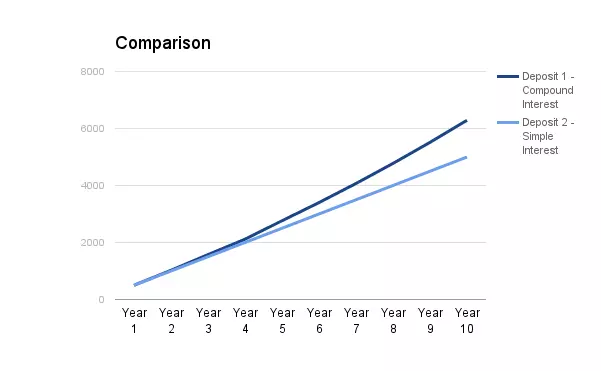From the graph above, we can see clearly the higher earnings through compound interest compared to simple interest. The difference is not too much upto the 4th year. This is because the interest accumulated over the years is added to the principal, thus making the principal significantly higher. From Year 5, there is a major difference in the interest earned. At the end of 10 years, Deposit 1 earns Rs. 6,288.95, while Deposit 2 earns Rs. 5,000. The difference between the two is Rs. 1,288.95.

## Compound Interest with Monthly Contributions

Compounding interest on fixed deposits where you are allowed to make monthly contributions can get a little tricky. For the amount invested during the compounding period, interest will be generated for the initial investment amount + monthly contributions. These deposits are rare but are an extremely good investment with whopping returns.

For example, Rs. 10,000 is the initial fixed deposit amount. The investor deposits Rs. 1,000 every month for 5 years. If the interest is compounded annually, then the interest will be as follows:

Period Investment Breakdown Investment + Interest Accumulated Interest Earned Total Value of Deposit
Year 1 10,000 + 12,000 22,000 1,100 23,100
Year 2 10000 + (12000 x 2) + 1,100 35,100 1,755 36,855
Year 3 10000 + (12000 x 3) + (1,100 +1,755) 48,855 2,442.75 51,297.75
Year 4 10000 + (12000 x 4) + (1,100 +1,755 + 2,442.75 ) 63.297.75 3164.87 66,462.64
Year 5 10000 + (12000 x 5) + (1,100 +1,755 + 2,442.75 + 3164.87 ) 78,461.75 3,923.13 82,385.77

Through this table, we can see that the interest earned is accumulated every year and added to the principal amount. The total money contributed by the investor is Rs. 10,000 initially, followed by Rs. 1,000 every month or Rs. 12,000 every year. The investor made a total contribution of Rs. 10,000 + Rs. 60,000. At the end of 5 years, the value of his deposit is Rs. 82,385.77. The total compound interest earned is Rs. 12,385.77.

## The Benefits of Compound Interest

Compound interest is your biggest friend when it comes to deposits and investments. Working in favor of investments, you stand to gain much more from the interest payable. But compound interest will be your worst enemy when it is calculated on your loan or other debt. You will end up paying significantly more interest on your loan. In terms of fixed deposits, compound interest is a great way of earning more on your investment. You earn much higher returns with compound interest on long term deposits. Compounding interest monthly, quarterly and half-yearly can spike your interest even higher. The benefits of compound interest can be listed as follows:

• Reinvestment - The interest earned will be reinvested into the same deposit.
• Higher value of the deposit - Compound interest leads to a higher value of the deposit. Upon maturity, your deposit will be more than a deposit with simple interest.
• Long-term savings - Compound interest deposits encourage long-term savings as the return on investment is much higher after 10 years or more.
• Increased Earnings - Options of compounding monthly, quarterly, and half-yearly increase the interest earned.

## Financial platforms where compound interest is applicable

Compound interest is used for both debit and credit aspects of the financial world. Listed below are some of the investments and credit options that use compound interest.

Investments

• Savings Accounts
• Fixed Deposits
• Recurring Deposits
• Other Certificates of Deposits
• Reinvested Dividend Stocks
• Retirement Funds

Debt

When it is used in case of deposits and investments, we stand to benefit. On the other hand, when compound interest is charged on loans and debt, the banks and lenders stand to gain.

## FAQs on Compund Interest Formula

1. Which kind of interest makes my investments grow faster – compound interest or simple interest?

Your investments will grow faster if it is calculated on the compound interest method as compared to the simple interest method because simple interest is calculated only on the principal amount whereas compound interest is calculated both on the principal and interest amount every year.

2. What is the schedule for compounding interest used for savings bank accounts?

The schedule for compounding interest used by savings bank accounts is on a daily basis.

3. Is compounding interest more beneficial for an investor or for a borrower?

Compounding interest is more beneficial to an investor than for a borrower.

## Disclaimer

Display of any trademarks, tradenames, logos and other subject matters of intellectual property belong to their respective intellectual property owners. Display of such IP along with the related product information does not imply BankBazaar's partnership with the owner of the Intellectual Property or issuer/manufacturer of such products.

## Compound Interest Formula Reviews

•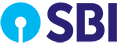"Good in FD service"
0.5 5.0/5 "Blown Away!"
I am having a savings account with State Bank of India hence i have selected the Fixed deposit investment scheme with them. They are providing the interest rate of 8%. I have invested the money 2 years back and there is a lockin period of 72 months.
, visakhapatnam
Reviewed on Mar 02, 2020
•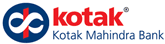"Safe and secure"
0.5 4.0/5 "Great!"
Kotak Mahindra bank is a reliable bank and good management thats the reason i have invested the fixed deposit scheme in this bank. They have provided me a interest rate of 7% and i have kept for the period of 1 year and 1 month. Overall, I'm satisfied with their services.
Reviewed on Mar 01, 2020
•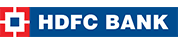"Safe and Secure"
0.5 5.0/5 "Blown Away!"
I have purchased HDFC Fixed deposit which I am renewing for every 13 months. They have given me an interest rate of 7% and There is no processing fee and there is no locking period as well. If I'm withdrawing FD amount before locking period, they are charging some amount.
Reviewed on Mar 01, 2020
•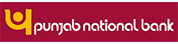"PNB Fixed Scheme is good"
0.5 5.0/5 "Blown Away!"
I went to the nearby branch for the Punjab National Bank for taking my fixed deposit as per my family members recommendation. I have invested the money of Rs. 50000 there was a lockin duration of 6 years when i withdraw the money, i got the money of Rs.1 lakhs. They have offered me a interest rate of 7%.
, jamshedpur
Reviewed on Feb 27, 2020
•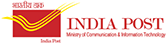"Good Service"
0.5 5.0/5 "Blown Away!"
I was having my savings account from Post office hence i have invested my money into Post office Fixed deposit scheme. I have invested the money of Rs. 25000. There is a lockin period of 10 years. I have invested the money in the year of 2011. Post office service is proper. Overall, I'm happy about the services.
, chennai
Reviewed on Feb 25, 2020
•"FD is not good with HDFC"
0.5 4.0/5 "Great!"
I have invested some money as a Fixed deposit in HDFC Bank and the interest rate is not that much great because they are giving interest as compared to others. I have invested the money of Rs. 3L and there is a locking period of 5 years. Its 7% interest rate. If they are increasing the interest rate, it would be fine. Since I do all my transactions with this bank, I have selected same for my FD as well.
, bangalore
Reviewed on Jan 31, 2020
•"Good support"
0.5 5.0/5 "Blown Away!"
I have chosen my fixed deposit scheme from State bank of India because I'm maintaining my salary account with them and they provided rate of interest is fine for me. I have invested the money of Rs. 2.75 lakhs. There is a locking period of 45 days. They are giving interest rate of 4.5%. Overall, I'm satisfied with their services.
, namakkal
Reviewed on Jan 18, 2020
•"Good App"
0.5 5.0/5 "Blown Away!"
I have been investing the money of Rs. 3 lakhs for the fixed deposit. I have selected Kotak mahindra bank for the FD because their net banking facility is very different when i compared to other. I can access everything regarding the account hence its easy to handle the transactions. I'm not sure about the interest rates. 7 months before, i have invested the money.
, bangalore
Reviewed on Jan 08, 2020
•"Excellent Services"
0.5 5.0/5 "Blown Away!"
Its because of the competitive interest rates, i have selected the Fixed deposit in Kotak Mahindra bank. I have invested the fixed deposit 1.5 years ago and there is a lockin period of 5 years. They have invested the amount of Rs. 7.5 % and the employees are helpful in the branch.
, mumbai
Reviewed on Jan 08, 2020
•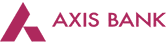"Good Savings scheme"
0.5 4.0/5 "Great!"
I have a Fixed deposit in Axis bank. I have invested the money of Rs. 10000 for two FDs. There is a lockin duration of 1 year. They are giving a interest rate of 7%. Through net banking, i have activated this Fixed deposit. There is no tax benefits available. Axis bank service has been good. Overall, I'm happy about their services.
, kolkata
Reviewed on Dec 31, 2019
•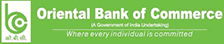"Good Scheme"
0.5 5.0/5 "Blown Away!"
Since last 15 years, i have a account with Oriental bank of commerce thats the reason i have invested the money in fixed deposit with the same bank. I have invested the amount of Rs. 11 lakhs. They are giving the interest rate of 6.5%. There is no locking period but i have chosen the duration of 1.5 years. There is no additional charges deducted for this fixed deposit.
, bulandshahr
Reviewed on Dec 03, 2019
•"Good Service"
0.5 4.0/5 "Great!"
Interest rate is very low in Indian post office Fixed deposit scheme because they are providing only 8.5%. I have chosen this office because of the child benefits. I have invested the money of Rs. 1.5 years. Its 3 years lock in period. Overall, I'm satisfied with the service provided by post office employees.
, parvathipuram
Reviewed on Dec 03, 2019
•"Good bank for Fixed Deposit"
0.5 4.0/5 "Great!"
I have invested the money in SBI for a fixed deposit and based on my wife recommendation i have chosen it. They are providing the interest rate of 7.25%. They have option to select the lock in period in that i have chosen 5 years. We had visited their branch and the response was good.
, mumbai
Reviewed on Nov 12, 2019
•"Good one"
0.5 4.0/5 "Great!"
Its easy accessible and to redeem so that i have invested the money in Fixed Deposit with HDFC BANK. I have invested the money of Rs. 2.5 lakhs and they have provided me a interest rate of 7.5%. There is no locking period. I didn't got any additional charges till now.
Reviewed on Nov 12, 2019
•"Safety deposit"
0.5 5.0/5 "Blown Away!"
I have paid the money of Rs. 4.5 lakhs as a fixed deposit in the Indian post office. For the safety purpose, i have chosen the post office account. They are offering me a interest rate of 7.9%. There is a lockin period of 5 years. If i withdraw the money in 1 year, they will reduce the interest rate of 2% and after 3 years, its 1%.
, jaipur
Reviewed on Nov 08, 2019
•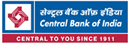"Good Bank"
0.5 4.0/5 "Great!"
Interest rates are less in Central Bank of India so I had taken a fixed deposit. I have invested the money of Rs. 1 lakh in my wife's name. Branch is near from my place so I have chosen this bank. Yearly once, I get the money of Rs. 6,765 for the interest. There is a locking period of 1 year.
, tirupur
Reviewed on Nov 06, 2019
•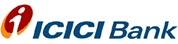"Customer centrice & User friendly app"
0.5 4.0/5 "Great!"
I am doing Fixed Deposit with ICICI BANK and its been around 5years. They haven't been paying good interest and its more or less same like other banks as I used to hold my salary account with ICICI, I have decided to be with one bank. There is no locking period and its flexible. They do give good support and the app is user friendly. Even deposit account can be opened through i-mobile or net banking itself.
, nasik
Reviewed on Oct 15, 2019
•"Good"
0.5 3.0/5 "Satisfactory"
I had a Fixed Deposit in State Bank of India, I have completed the tenure before 3months ago. I have no issues with the customer service. They are given me 7% interest rate for 2years. There is no profit and processing fee. I got an online access, where I can check the interest rate anytime on the YONO app or else SBI online.
, tirupati
Reviewed on Oct 15, 2019
•"Interest charges are good. "
0.5 3.0/5 "Satisfactory"
I have invested some money through FD. My salary account is in SBI so i have a zero ATM charges and the limit is not actually set by SBI. They do have short term FDs which is pretty good. I have invested the money of Rs. 60000 and the interest rate is 10.01% for the tenure period of 16 months. There is no lockin period. There is no hassle to getting the money back.
•